International
Tables for
Crystallography
Volume F
Crystallography of biological macromolecules
Edited by M. G. Rossmann and E. Arnold

International Tables for Crystallography (2006). Vol. F, ch. 11.4, pp. 227-228   | 1 | 2 |

## Section 11.4.3. Autoindexing

Z. Otwinowskia* and W. Minorb

aUT Southwestern Medical Center at Dallas, 5323 Harry Hines Boulevard, Dallas, TX 75390-9038, USA, and bDepartment of Molecular Physiology and Biological Physics, University of Virginia, 1300 Jefferson Park Avenue, Charlottesville, VA 22908, USA
Correspondence e-mail:  zbyszek@mix.swmed.edu

### 11.4.3. Autoindexing

| top | pdf |

Among the number of autoindexing algorithms proposed (Vriend & Rossmann, 1987; Kabsch, 1988; Kim, 1989; Higashi, 1990; Leslie, 1993), the method based on periodicity of the reciprocal lattice tends to be the most reliable (Otwinowski & Minor, 1997; Steller et al., 1997).

Autoindexing starts with a peak search, which results in the set of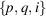triplets, where i is the number of the image in which the peak with position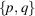was found. The program takes advantage of the fact that for any rotation matrix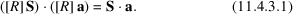When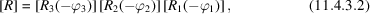equation (11.4.3.1) applied to equation (11.4.2.2) becomes: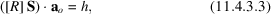where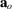is a three-dimensional vector with as yet unknown components. Note that the matrix [R] represents crystal rotation when the crystal is in the diffraction condition defined by the existence of the solution to equations (11.4.2.1)–(11.4.2.4), described by vector S. For data collected in the wide oscillation mode2 the angle at which diffraction occurs is not known a priori; however, it can be approximated by the middle of the oscillation range of the image. Combining the peak positionwith equations (11.4.2.5) and (11.4.2.8) provides an estimate of the vector S. So, we expect that equation (11.4.3.3) and similar equations for k and l are approximately (owing to approximation and experimental errors) satisfied. The purpose of autoindexing is to determine the unknown vectors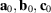and the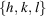triplet for each peak. To accomplish this, three equations (11.4.3.3) for each peak must be solved. DENZO introduced a method based on the observation that the maxima of the function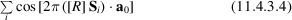are the approximate solutions to this set of equations (11.4.3.3). To speed up the search for all significant maxima, a two-step process is used. The first step is the search for maxima of function (11.4.3.3) on a three-dimensional uniform grid, made very fast owing to the use of a fast Fourier transform (FFT) to evaluate function (11.4.3.4). Function (11.4.3.4) is identical to structure-factor calculations in the space group P1, which allows the use of the crystallographic FFT. Because the maxima at the grid points (HKL uses a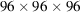grid) only approximate the maxima of function (11.4.3.4), the vectors resulting from a grid search are optimized by the Newton method. Function (11.4.3.4) has maxima not only for basic periodic vectors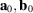and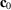, but also for any integer linear combination of them. Any set of three such vectors with a minimal nonzero determinant can be used to describe the crystal lattice. Steller et al. (1997) describe the algorithm that finds the most reliable set of three vectors. This set needs to be converted to the one conventionally used by crystallographers, as defined in IT A (2005).

To generate the conventional solution, two steps are used. Step 1 finds the reduced primitive triclinic cell. IT A provides the algorithm for this step. Subsequently, step 2 finds conventional cells in Bravais lattices of higher symmetry.

#### 11.4.3.1. Lattice symmetry

| top | pdf |

The relationship between a higher-symmetry cell and the reduced primitive triclinic cell can be described by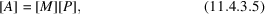where [A] and [P] are matrices of the type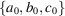, with [P] representing the reduced triclinic primitive cell, and [M] is one of the 44 matrices listed in IT A .3 If [A] is generated using equation (11.4.3.5) from an experimentally determined [P], owing to experimental errors it will not exactly satisfy the symmetry restraints. DENZO introduced a novel index that helps evaluate the significance of this violation of symmetry. This index is based on the observation that from [A] one can deduce the value of the unit cell, apply symmetry restraints to the unit cell and calculate any matrix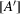for the unit cell that satisfies these symmetry restraints. If [A] satisfies symmetry restraints, the matrix [U], where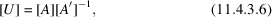will be unitary and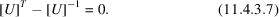The index of distortion printed by DENZO is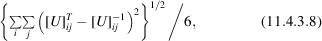where i and j are indices of thematrix [U].

The value of this index increases as additional symmetry restraints are imposed, starting from zero for a triclinic cell. Autoindexing in DENZO always finishes with a table of distortion indices for 14 possible Bravais lattices, but does not automatically make any lattice choice.

#### 11.4.3.2. Lattice pseudosymmetry

| top | pdf |

The cell-reduction procedure cannot determine lattice symmetry, since it cannot distinguish true lattice symmetry from a lattice accidentally having higher symmetry within experimental error (e.g. a monoclinic lattice with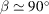is approximately orthorhombic). If one is not certain about the lattice symmetry, the safe choice is to assume space group P1, with a primitive triclinic lattice for the crystal, and to check the table again after the refinement of diffraction-geometry parameters. A reliable symmetry analysis can be done only by comparing intensities of symmetry-related reflections, which is done later in SCALEPACK or another scaling program.

#### 11.4.3.3. Data-collection requirements

| top | pdf |

The total oscillation range has to cover a sufficient number of spots to establish periodicity of the diffraction pattern in three dimensions. It is important that the oscillation range of each image is small enough so that the lunes (rings of spots, all from one reciprocal plane) are resolved. One should note that the requirement for lune separation is distinct from the requirement for spot separation. If lunes overlap, spots may have more than one index consistent with a particular position on the detector.

The autoindexing procedure described above is not dependent on prior knowledge of the crystal unit cell; however, for efficiency reasons, the search is restricted to a reasonable range of unit-cell dimensions, obtained, for example, from the requirement of spot separation. In DENZO, this default can be overridden by the keyword `longest vector', but the need to use this keyword is a sign of a problem that should be fixed. Either the defined spot size should be decreased or data should be recollected with the detector further away from the crystal.

#### 11.4.3.4. Misindexing

| top | pdf |

Autoindexing is sensitive to inaccuracy in the description of the detector geometry. The specified position of the beam on the detector should correspond to the origin of the Bragg-peaks lattice (Miller index 000). Autoindexing will shift the origin of the lattice to the nearest Bragg lattice point. An incorrect beam position will result in the nearest Bragg lattice point not having the index 000. In such a situation, all reflections will have incorrectly determined indices. Such misindexing can be totally self-consistent until the intensities of symmetry-related reflections are compared. This dependence of the indexing correctness on the assumed beam position is the main source of difficulties in indexing (Gewirth, 1996; Otwinowski & Minor, 1997). The beam position has to be precise, as the largest acceptable error is one half of the shortest distance between spots.

Indexing limited to determining h, k, l triplets is not very sensitive to other detector parameters. Errors by a degree or two in rotation or by 10% in distance are unlikely to produce wrong values of h, k and l. Sometimes even a very large error, such as the distance being too large by a factor of 5, will still produce the correct h, k, l triplets. The detector position error will be compensated by an error in the lattice determined by autoindexing. For this reason, the accuracy of the lattice is not a function of the autoindexing procedure, but depends mainly on the accuracy of the detector description. By the same token, the distortion of the lattice also depends on the accuracy of the detector parameters.

#### 11.4.3.5. Twins

| top | pdf |

Special care has to be taken if more than one crystal contributes to the diffraction image. When there is a large disproportion between volumes (e.g. the presence of a satellite crystal), autoindexing may work without any modifications. In the case of similar volumes, the manual editing of weaker reflections and resolution cuts can make the proportion of reflections from one crystal in the peak-search list large enough for the autoindexing method to succeed. If the crystals have a similar orientation, using only very low resolution data may be the right method. In the case of twinned crystals, autoindexing sometimes finds a superlattice that results in integer indices simultaneously for both crystals. In such a case, DENZO solves the problem of finding the best three-dimensional lattice that incorporates all of the observed peaks. Unfortunately, for a twinned crystal, this is a mathematically correct solution to an incorrectly posed problem.

### References

International Tables for Crystallography (2005). Vol. A. Space-group symmetry, edited by Th. Hahn. Heidelberg: Springer.Google Scholar
Gewirth, D. (1996). HKL manual. 5th ed. Yale University, New Haven, USA.Google Scholar
Higashi, T. (1990). Auto-indexing of oscillation images. J. Appl. Cryst. 23, 253–257.Google Scholar
Kabsch, W. (1988). Evaluation of single-crystal X-ray diffraction data from a position sensitive detector. J. Appl. Cryst. 21, 916–924.Google Scholar
Kim, S. (1989). Auto-indexing oscillation photographs. J. Appl. Cryst. 22, 53–60.Google Scholar
Leslie, A. (1993). Autoindexing of rotation diffraction images and parameter refinement. In Proceedings of the CCP4 study weekend. Data collection and processing, 29–30 January, edited by L. Sawyer, N. Isaac & S. Bailey, pp. 44–51. Warrington: Daresbury Laboratory.Google Scholar
Otwinowski, Z. & Minor, W. (1997). Processing of X-ray diffraction data collected in oscillation mode. Methods Enzymol. 276, 307–326.Google Scholar
Steller, I., Bolotovsky, R. & Rossmann, M. G. (1997). An algorithm for automatic indexing of oscillation images using Fourier analysis. J. Appl. Cryst. 30, 1036–1040.Google Scholar
Vriend, G. & Rossmann, M. G. (1987). Determination of the orientation of a randomly placed crystal from a single oscillation photograph. J. Appl. Cryst. 20, 338–343.Google Scholar